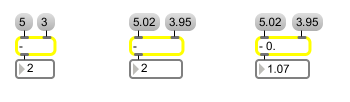# -

Subtract two numbers, output the result

## Description

- takes any two given numbers, subtracts them, and then outputs the result.

## Arguments

Name Type Opt Description
initial-value int or float opt Sets the initial value, to be subtracted from a number received in the left inlet. A float argument causes the numbers to be subtracted as floats.

## Messages

 bang In left inlet: Performs the subtraction with the numbers currently stored. If there is no argument, - initially holds 0. int input [int] In left inlet: The number in the right inlet is subtracted from the number, and the result is sent out the outlet. (inlet1) value-subtracted-from [int] In right inlet: The number is stored, to be subtracted from, by a number received in the left inlet. float input [float] Converted to int, unless - has a float argument. set set-input [int] Sets the number to be subtracted without causing output (bang will output it). list input [list] In left inlet: The first number is subtracted from the second number, and the result is sent out the outlet.

## Output

float: Only if there is an argument with a decimal point.
int: The difference between the two numbers received in the inlets.

## ExamplesSubtracted as ints... Floats are truncated before subtraction... unless there is a float argument